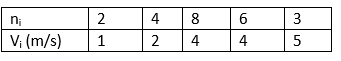# Online Test for Class 11 Physics Kinetic Theory of Gases

Welcome to this Free Online Mock Test Class 11 Physics Kinetic Theory of Gases. This test contains MCQs for Chapter 11 Kinetic Theory of Gases with Answers for Class 11 Physics. The MCQ questions were created using the most recent exam format. Practicing these questions would allow students to check their knowledge and have a good grasp of the concepts.

## Class 11 Physics Kinetic Theory of Gases Online Test

General Instructions

1. Your test contains multiple-choice questions with only one answer type questions. There are a total of 20 questions
2. This is a 30 min test. Please make sure you complete it in stipulated time
3. You can finish this test any time using the 'Submit' button.

1. The temperature of a gas is held constant, while its volume is decreased. The pressure exerted by its volume is decreased. The pressure exerted by the gas on the walls of the container increases, because its molecules
2. A jar has a mixture of hydrogen gases in the ratio of 1 : 5. The ratio of mean kinetic energies of hydrogen and oxygen molecules is
3. The mean free path of collision  of gas molecules varies with its diameter (d) of the molescules as
4. The r.m.s of speed of a group of 7 gas molecules having speed  ( 5,3,1,0,-1,-3,-5) m/s is
5. The number of degrees of freedom for a diatomic gas molecule is
6. Relation between Pressure P and average Kinetic energy E per unit volume of a gas is
7. The molar specific heat of a gas as given from the kinetic theory is 5R/2. If it is not specified whether it is Cp or Cv, one could conclude that the molecules of the gas
8. The absolute zero is the temperature at which
9. If a gas has n degrees of freedom , then ratio of specific heats of gas is
10. Three perfect gasses at absolute temperatures $T_1$, $T_2$, and $T_3$ are mixed. The masses of molecules are $m_1$,$m_2$ and $m_3$ and the number of molecules of the gasses is $n_1$, $n_2$, and $n_3$ respectively then assuming no loss of energy, the final temperature of the mixture will be
11. In the following questions, a statement of assertion is followed by a statement of reason. You are required to choose the correct one out of the given four responses and mark it as
(a) If both assertion and reason are true and the reason is the correct explanation of the assertion.
(b) If both assertion and reason are true but the reason is not the correct explanation of the assertion.
(c) If the assertion is true, but the reason is false.
(d) If both assertion and reason are false.
(e) If the reason is true but the assertion is false.

Assertion: In the free expansion of an ideal gas, the entropy increases.
Reason: Entropy increases in all-natural processes.
12. In the following questions, a statement of assertion is followed by a statement of reason. You are required to choose the correct one out of the given four responses and mark it as
(a) If both assertion and reason are true and reason is the correct explanation of the assertion.
(b) If both assertion and reason are true but reason is not correct explanation of the assertion.
(c) If assertion is true, but reason is false.
(d) If both assertion and reason are false.
(e) If reason is true but assertion is false.

Assertion: Air pressure in a car tire increases during driving
Reason: Absolute zero temperature is not zero energy temperature
13. In the following questions, a statement of assertion is followed by a statement of reason. You are required to choose the correct one out of the given four responses and mark it as
(a) If both assertion and reason are true and reason is the correct explanation of the assertion.
(b) If both assertion and reason are true but reason is not correct explanation of the assertion.
(c) If assertion is true, but reason is false.
(d) If both assertion and reason are false.
(e) If reason is true but assertion is false.

Assertion: The root mean square  and most probable speeds of the molecules in a gas are the same
Reason: The maxwell distribution for the speed to molecules  in a gas is symmetrical
14. In the following questions, a statement of assertion is followed by a statement of reason. You are required to choose the correct one out of the given four responses and mark it as
(a) If both assertion and reason are true and reason is the correct explanation of the assertion.
(b) If both assertion and reason are true but reason is not correct explanation of the assertion.
(c) If assertion is true, but reason is false.
(d) If both assertion and reason are false.
(e) If reason is true but assertion is false.

Assertion: Vibrational energy of diatomic molecule corresponding to each degree of freedom is $k_BT$.
Reason: For every molecule, the vibrational degree of freedom is 2.
15. You have the following group of particles,$n_1$ represents the number of molecules with speed $v_1$?Find the average speed
16. Find the rms speed
17. Find the most probable speed?
18. The critical volume of a gas obeying van der Waals’ equation is
19. When you make ice cubes, the entropy of water
20. Which of the following is not a state function?

Subscribe
Notify ofThis site uses Akismet to reduce spam. Learn how your comment data is processed.